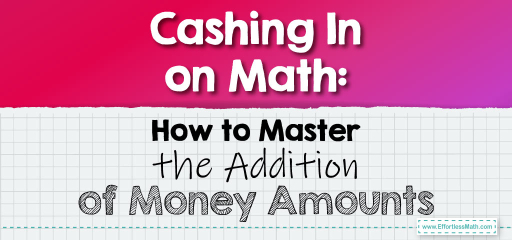# Cashing In on Math: How to Master the Addition of Money Amounts

Money is a practical application of decimals, and adding money amounts is a skill we use almost daily. Whether you're calculating expenses, summing up savings, or just splitting a bill, understanding how to accurately add money amounts is essential. Let's delve into the process.The Process Explained: When adding money amounts, it’s crucial to line up the decimal points. This ensures that dollars are added to dollars and cents to cents.

### Example 1:

Add $12.45 and$8.30.

Solution Process:

Line up the decimal points and add.

$12.45 +$8.30

________

$20.75 Answer: The total is$20.75.

The Absolute Best Book for 5th Grade Students

### Example 2:

Add $15.20,$9.80, and $5.00. Solution Process: Line up the decimal points and add.$15.20

+$9.80 +$5.00

________

$30.00 Answer: The total is$30.00.

Adding money amounts is more than just a mathematical exercise; it’s a life skill. By ensuring that you line up the decimal points correctly and add them systematically, you can avoid errors and ensure accurate results. Whether you’re budgeting, shopping, or planning an event, this skill will always come in handy. So, the next time you’re faced with adding money amounts, tackle it with precision and confidence, knowing you’ve got the math on your side!

### Practice Questions:

1. Add $4.50 and$3.25.

2. Sum up $7.75,$2.25, and $10.00. 3. Add$6.60 and $5.40. 4. Calculate the total of$12.12, $8.88, and$9.00.

A Perfect Book for Grade 5 Math Word Problems!

1. $7.75 2.$20.00

3. $12.00 4.$30.00

The Best Math Books for Elementary Students

### What people say about "Cashing In on Math: How to Master the Addition of Money Amounts - Effortless Math: We Help Students Learn to LOVE Mathematics"?

No one replied yet.

X
51% OFF

Limited time only!

Save Over 51%

SAVE $15 It was$29.99 now it is \$14.99